Maths Brain Teasers 104 :: Can You - Complete The Triangle?

in STEMGeeks2 months ago (edited)

Hey All; @StemGeeks Mathematician;

Without further, ado, let's get to our puzzle for today:: Maths Brain Teasers 104 :: Can You - Complete The Triangle?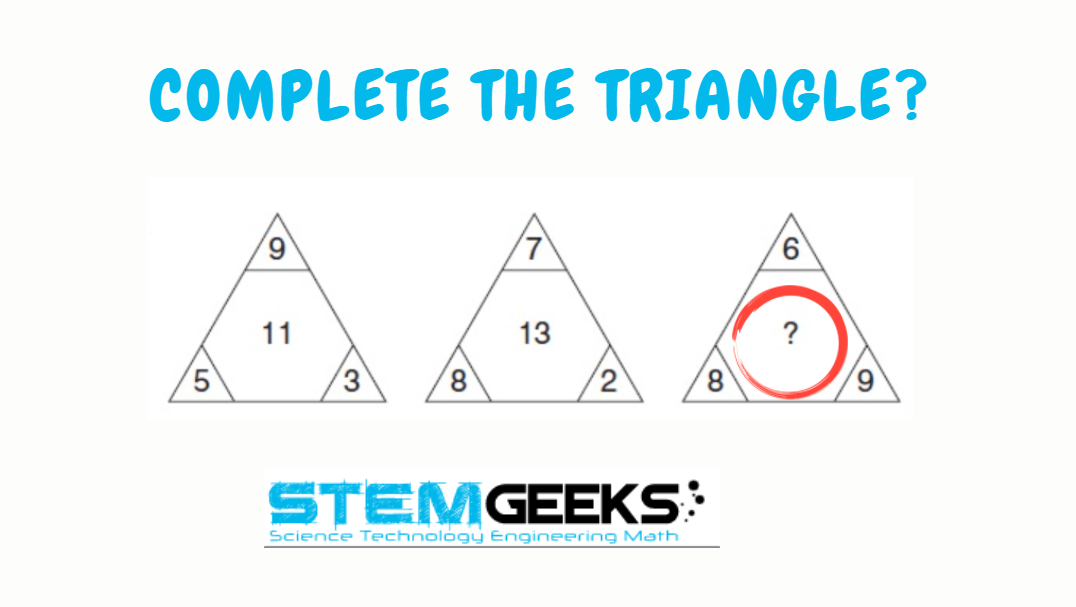Have a close look at the image given and see if you can decode the logic given in the image to complete the puzzle. Try finding out the right NUMBER that Simplifies or Decodes the logic to the given puzzle. Replace the question marks with the right numbers so that it completes the series.

There is logic to resolve the puzzle; try finding out the logic and it should be resolved in seconds.. Guesswork isn't going to help...

With that, I'll leave you all with the Maths Brain Teasers 104 :: Can You - Complete The Triangle? Good luck solving the puzzle...

Maths Brain Teasers 103 :: Can You - Complete The Sequence? - Solved with Explanation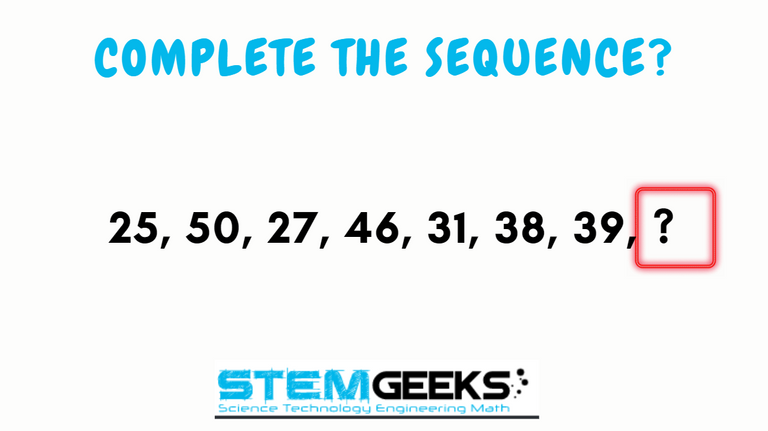Let's have a look at the Step by Step instructions to Solve the Maths Brain Teasers 103 :: Can You - Complete The Sequence?

If you carefully look at the Image in question; You would have noticed the following pattern is being followed::

The logic was::

2, 4, 8 etc and

The second sequence decreases by::

4, 8, 16 etc..

Really liked the explanation given on paper by @adedayoolumide ; clear and precise to the point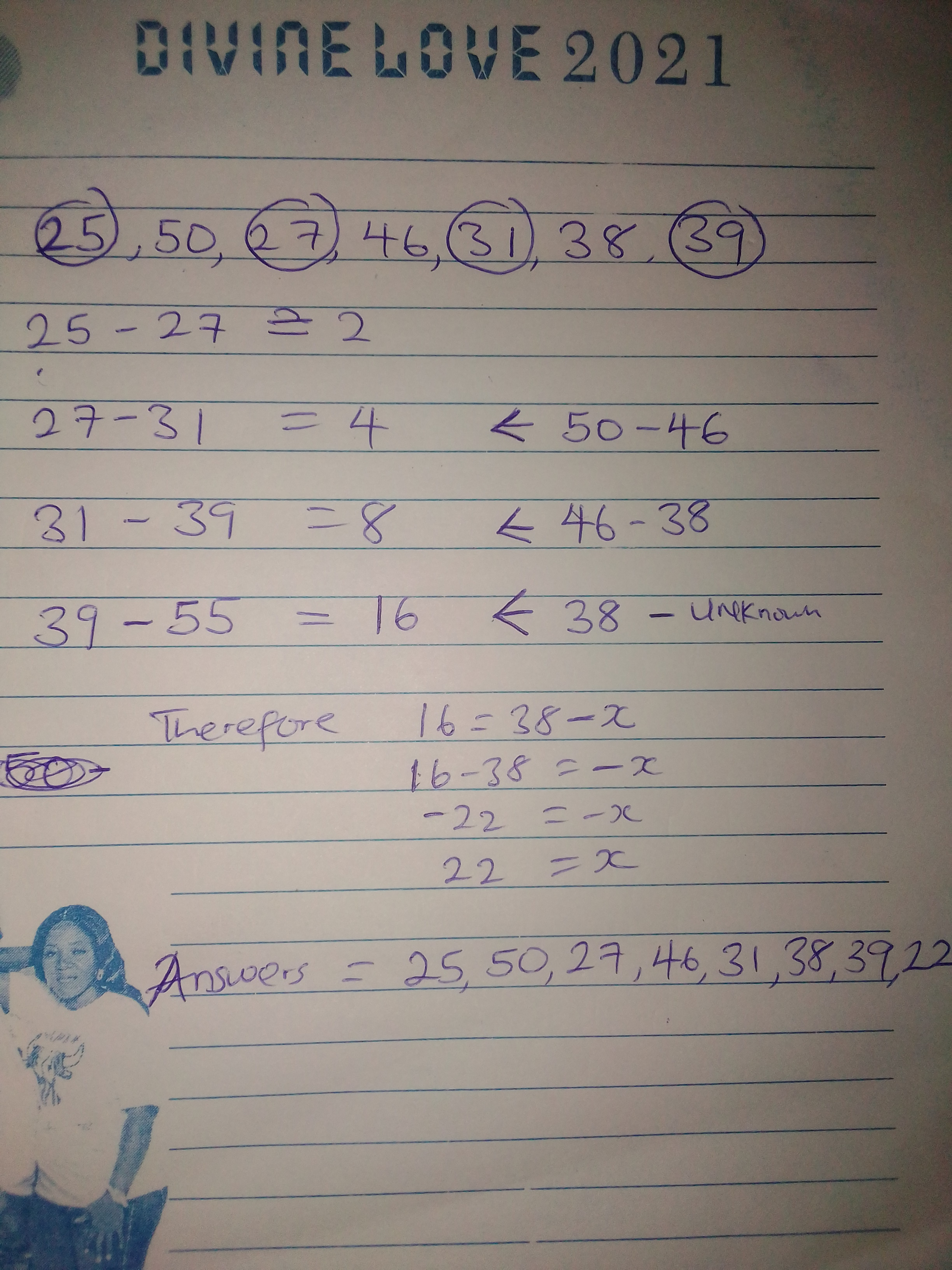Hence the answer to the Puzzle is Number:: 22

STEM token GiveAway

I'll be again doing a giveaway of STEM tokens to the lucky random winner with the correct answer. For the last contest, which was Maths Brain Teasers 103 :: Can You - Complete The Sequence?.

We had only 8 entries and I am happy to see the engagement. The highest number of entries we got this time. At the same time, it's great to see the detailed answers provided by everyone as to how they arrived at the solution of the puzzle.. Well done guys.. Way to GO... Now the results part; all entries apart from two entries provided the right answer. So I am going to use a random comment picker tool to pick one Winner. And the Winner is::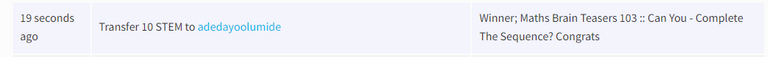Congratulations @adedayoolumide - You WIN yourself 10 STEM tokens. You should be having the rewards in your STEM Wallet Soon.

Math Quote for the Day::

Here is the motivation to solve the Maths Equation Puzzle?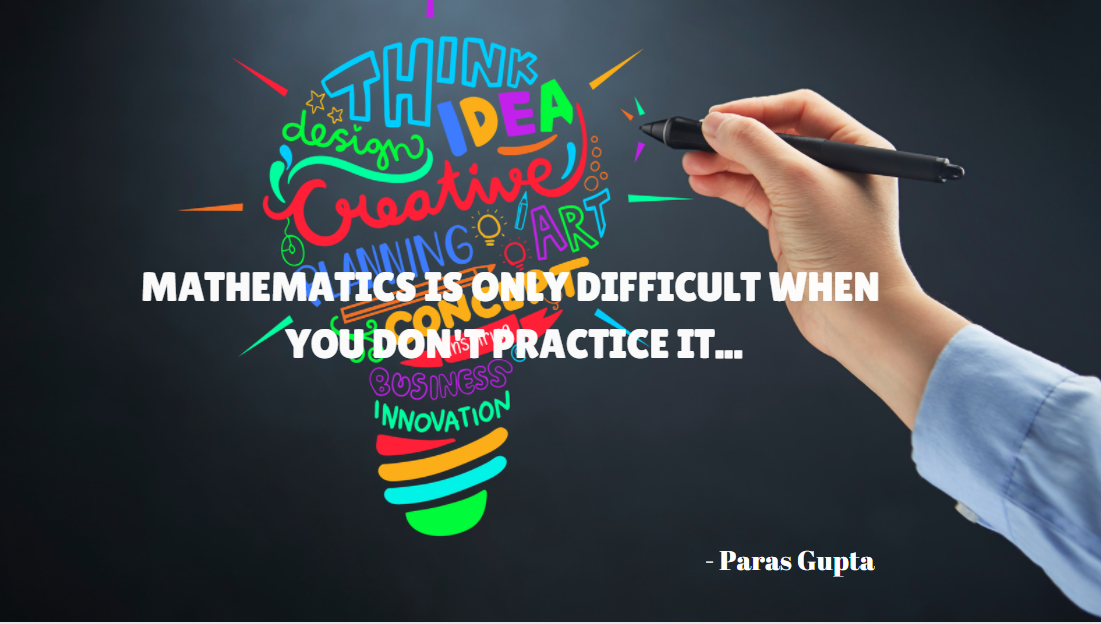If you like my work, then please spread the Word.. that we do have the Math Brain Teasers competition here @StemGeeks platform. Reblog is much appreciated.

Best RegardsFind Me on the Other Social Media Platforms::PS:: All the Maths Brain Teasers; are made by me using the Pro Canva License Version

Sort:

9+5=14-9=11
8+7=15-2=13
8+6=14-9=5

9+5-11= 3
8+7-13= 2
8+6-5= 9

5-3=2, 2+9=11
8-2=6,6+7=13
8-9=-1,-1+6=5

9+5=14-3=11
8+7=15-2=13
8+6=14-9=5

Using additional and substraction method to get the number in the middle.
9+5-3= 11
7+8-2= 13
6+8-9= 5

Thank you for the token

2 months ago (edited)

It's actually Summing the right two triangle value and subtracting the left most one.

9+5-3=14
And 8+7-2=13,